Whale provides multiple parallel strategies, such as data parallelism, pipeline parallelism, model parallelism based on layer splitting, model parallelism based on operator splitting, and various hybrid parallel strategies. This topic describes the logic of and methods for implementing the parallel strategies in Whale. You can follow the instructions in this topic to use Whale for distributed training.

## Data parallelism

• Background

When a large amount of application data is used to train a machine learning model, data parallelism is a common practice to speed up distributed training. Data parallelism is one of the basic parallelization methods that are supported by Whale and is most widely used for distributed training.

• Definition

In data parallelism, training data shards are distributed to different nodes and computed by using the same logic.

• Implementation logic
In this example, a ResNet50 model is used to describe how to implement data parallelism. The following figure shows the model.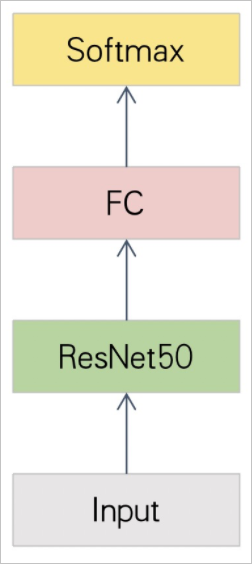In data parallelism, each graphics processing unit (GPU) runs an independent model replica. Each model replica reads different training data, as shown in the following figure.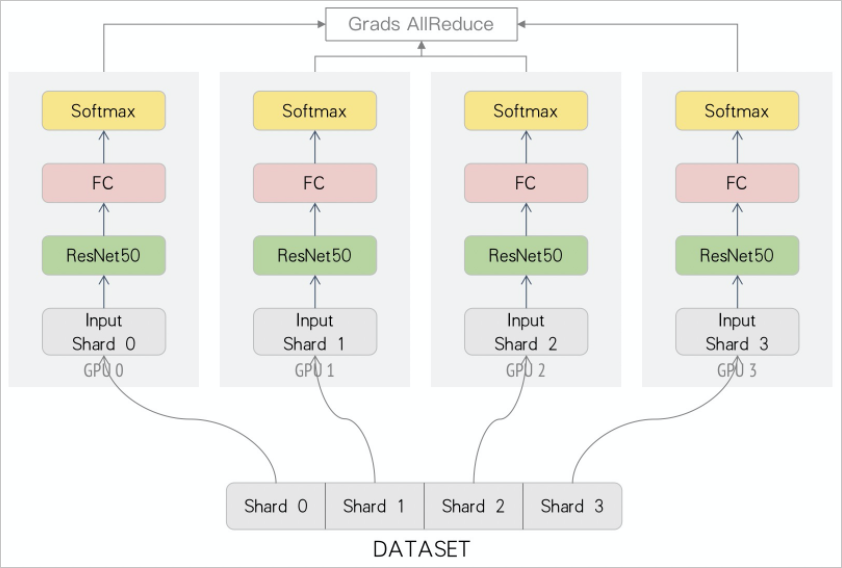Each iteration consists of three steps:
1. Calculate the loss for each model replica to obtain a gradient by using the model parameters, such as weight, that are updated in the previous iteration.
2. Perform the reduction operation on the gradients of all model replicas and apply the reduced gradient to all model replicas. In this example, run the ReduceSum function to reduce the gradients of all workers.
3. Use the reduced gradient to update the values of model parameters such as weight.
• Data parallelism in Whale
To implement data parallelism in Whale, you can use the default cluster layout method and add the model code to the scope code. The following code provides an example. For more information about the complete code of data parallelism, visit simple_data_parallel.py.
``````import whale as wh
wh.init()
with wh.cluster():
with wh.replica():
ResNet50_Model_Defination()``````

## Model parallelism

• Background
Development in deep neural networks significantly improves the model performance in fields such as natural language processing (NLP) and computer vision (CV), and also increases the size of model parameters. For example, the number of the parameters that were used by the GoogleNet model for ImageNet classification in 2014 is 4 million. The number of the parameters that were used by the Squeeze-and-Excitation Networks model for ImageNet classification in 2018 is 145.8 million. The parameter size increases by 35 times. The number of the parameters that are used by the Turing-NLG model even reaches 17 billion. The rapid growth of model parameter size leads to the following issues:
• The communication traffic of gradient synchronization in distributed training increases. Limited GPU memory results in a small batch size. This increases the proportion of the communication for training and weakens the scalability of distributed training.
• A smaller batch size increases the instability of model training and depreciates the convergence performance.
Model parallelism is applicable to large-scale models or models with large-scale parts. You cannot train these models or significantly accelerate the training of these models by solely using data parallelism. For example, you can apply model parallelism to the following models:
• The GPT2 and T5 models in NLP. These models have an ultra-large parameter size. They consume a large amount of GPU memory and cannot be trained by solely using data parallelism.
• The BertLarge model in NLP. This model has a large parameter size. Feature mapping exists between layers in the model. The traffic for feature mapping communication is much less than that for weight communication.
• The VGG-16 model. The GPU memory of this model differs between layers. Therefore, if you solely use data parallelism to train this model, a large amount of GPU memory is wasted.
• Definition

In model parallelism, different computing devices, such as GPUs and CPUs, perform computing at different layers of a network model. For example, the different network layers of a neural network model are distributed to different devices.

• Implementation logic
In this example, the BertLarge model is used. The layers of the BertLarge model are distributed to different GPUs. This reduces the occupied memory of each GPU and increases the batch size. In addition, activation communication is used to replace gradient synchronization. This greatly reduces the communication traffic between GPUs. The following figure shows model parallelism.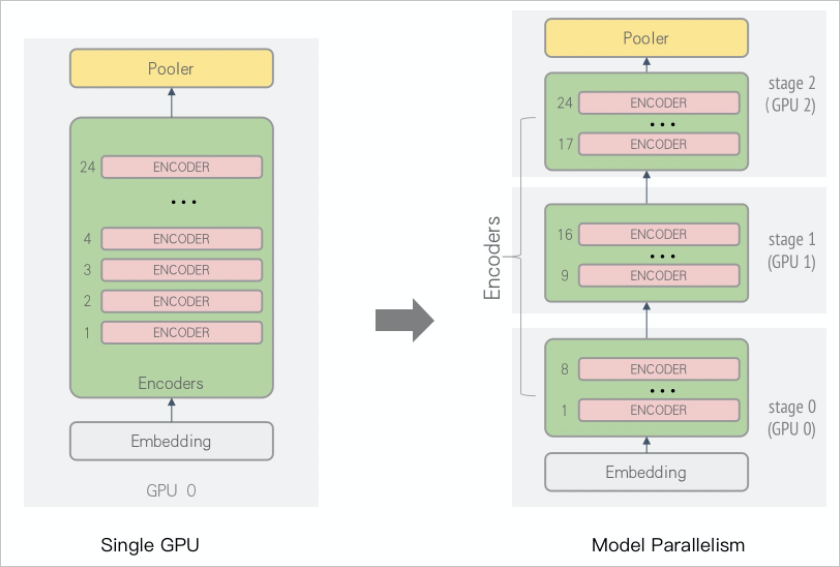In data parallelism, all the gradients of BertLarge need to be synchronized in each iteration. This consumes about 1.2 GB traffic. In addition, the model is large in size. In an environment of 16 GB V100, gradient synchronization is required each time two samples are calculated. At the same time, you must consider other factors such as the sequence length and embedding size. A high proportion of communication results in poor performance. A small batch size leads to instable model training and poor convergence. In model parallelism, only 27 MB of activation data needs to be communicated for each iteration. No gradient synchronization is required. In addition, the batch size is greatly increased, which can improve the training stability and convergence performance.
• Model parallelism in Whale
To implement model parallelism in Whale, you must configure the cluster layout and add different layers of your model to different stages. In this example, the BertLarge model is used. The following two code blocks provide an example on a single-GPU model and an example on model parallelism. For more information about the complete code of model parallelism, visit train_bert_model.py.
• Single-GPU model:
``````embedding_output = embedding(inputs)
encoder_output = encoder(embedding_output)
pooler_output = pooler(encoder_output)``````
• Model parallelism:
``````import whale as wh
cluster = wh.cluster()

with cluster:
with wh.stage():
embedding_output = embedding(inputs)
encoder_output = encoder_layer_1_8(embedding_output)

with wh.stage():
encoder_output = encoder_layer_9_16(embedding_output)

with wh.stage():
encoder_output = encoder_layer_17_24(embedding_output)
pooler_output = pooler(encoder_output)``````
Note To accelerate training, you can use model parallelism together with pipeline parallelism.

## Pipeline parallelism

• Background

In model parallelism, the entire model is split into multiple stages and distributed to different GPUs for computing. Stages depend on each other. As a result, GPUs for different stages cannot be used at the same time. This causes a waste of GPU computing power. You may not need to split a model into a large number of layers, but you may need to use a large amount of data that is generated in your business to train a model. More data requires higher training performance. To accelerate training and improve convergence performance, you must use more computing resources for parallel computing.

To resolve the issues of model parallelism, Whale provides hybrid strategies to improve GPU utilization and the scalability of distributed training. You can use model parallelism together with pipeline parallelism, or together with pipeline parallelism and data parallelism.

• Definition

Pipeline parallelism is an auxiliary parallel strategy that is usually used with model parallelism. In pipeline parallelism, different devices such as GPUs and CPUs, receive different batches of data and perform computing at different network layers. The data contains raw training data, activation data, and error data.

• Implementation logic
Applicable parallel strategies may vary with the scenario. In this example, the BertLarge model is used. The activation process, GPU memory, and number of flops are almost the same at each layer, regardless of the bandwidth and hardware topology of the model. Layer splitting is more prone to be affected by the load balancing of GPU memory and computing power. In this case, you can use a hybrid strategy of model parallelism, pipeline parallelism, and data parallelism to optimize the performance of BertLarge. The hybrid strategy can be implemented based on the following logic:
1. Model parallelism
You can split a large-scale BertLarge model into multiple layers and distribute the layers to different GPUs for computing. This reduces the occupied memory of each GPU and increases the batch size. In addition, activation communication is used to replace gradient synchronization. This greatly reduces the communication traffic between GPUs. The following figure shows model parallelism.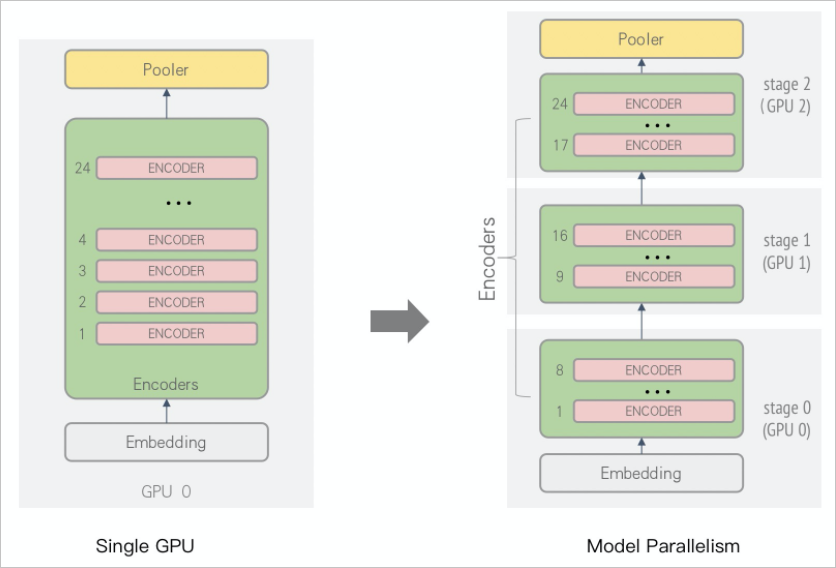2. Pipeline parallelism
Assume that you solely use model parallelism that is based on layer splitting. Only one GPU runs at a time and other GPUs are idle, as shown in the following figure.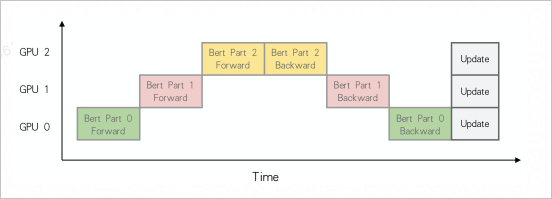In this case, you can use pipeline parallelism together with model parallelism to improve the utilization of other GPUs. Each GPU starts to train the next batch of data immediately after the current data batch is trained. The following figure shows the implementation logic. You can optimize the implementation logic in actual use.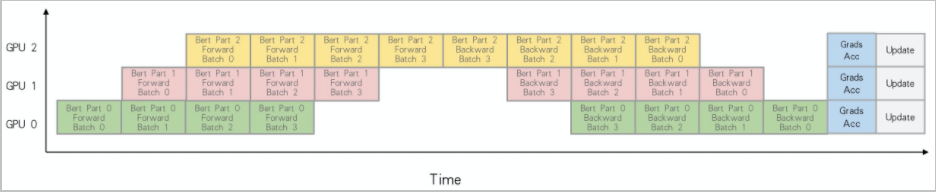3. Data parallelism
You cannot split a model into infinite layers. Excessive layers may depreciate model performance. Therefore, you must figure out how to use more GPUs to accelerate training. To use a large amount of data for training, you can implement data parallelism by using more GPUs. In this case, make sure that the split model layers for model parallelism are distributed to different servers. Gradient synchronization for data parallelism, which consumes about 1.2 GB traffic, is performed on a server. A TCP network is used for activation communication between the model layers, which consumes about 27 MB traffic. This way, you can efficiently utilize the NVLink or Peripheral Component Interconnect Express (PCI-e) bandwidth between GPUs and reduce the costs of gradient synchronization. Assume that each server has four GPUs and data parallelism is implemented for four replicas of the BertLarge model. The following figure shows the logic of model splitting.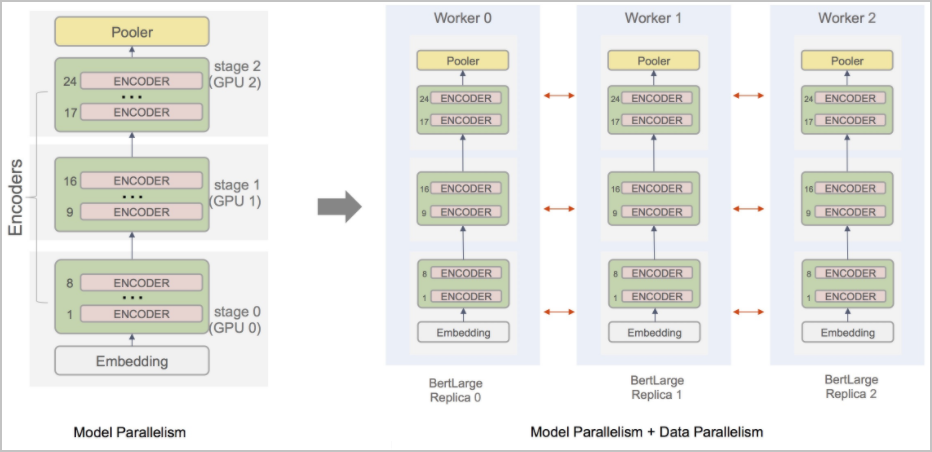The model parallelism, pipeline parallelism, and data parallelism strategies are combined by using the simple and flexible API operations of Whale. This accelerates the training of the BertLarge model.
• Pipeline parallelism in Whale
To implement model parallelism in Whale, you must configure the cluster layout and add different layers of your model to different stages. In this example, the BertLarge model is used. The following two code blocks provide an example on a single-GPU model and an example on pipeline parallelism. For more information about the complete code of pipeline parallelism, visit train_bert_model.py.
• Single-GPU model:
``````embedding_output = embedding(inputs)
encoder_output = encoder(embedding_output)
pooler_output = pooler(encoder_output)``````
• Pipeline parallelism:
``````import whale as wh
# A server with three GPUs is used.
cluster = wh.cluster()

with cluster:
with wh.pipeline(num_micro_batch=5):
with wh.stage(): # stage 0
embedding_output = embedding(inputs)
encoder_output = encoder_layer_1_8(embedding_output)

with wh.stage(): # stage 1
encoder_output = encoder_layer_9_16(embedding_output)

with wh.stage(): # stage 2
encoder_output = encoder_layer_17_24(embedding_output)
pooler_output = pooler(encoder_output)``````
• A hybrid of pipeline parallelism and data parallelism
``````import whale as wh
# Three servers with four GPUs are used.
# Data parallelism on four replicas is performed for each stage.
cluster = wh.cluster(layout={"average": 4}) # You can also set the layout parameter to row.

with cluster:
with wh.replica():
with wh.pipeline(num_micro_batch=5):
with wh.stage(): # stage 0
embedding_output = embedding(inputs)
encoder_output = encoder_layer_1_8(embedding_output)

with wh.stage(): # stage 1
encoder_output = encoder_layer_9_16(embedding_output)

with wh.stage(): # stage 2
encoder_output = encoder_layer_17_24(embedding_output)
pooler_output = pooler(encoder_output)``````

## Operator splitting

• Background
Deep learning models are becoming more complex and data parallelism is insufficient for task training. For example, data parallelism has poor performance in training models in a distributed manner and cannot provide sufficient memory for storage in a single GPU. Therefore, operator splitting needs to be implemented to accelerate model training in a distributed manner. Operator splitting is applicable to large-scale models or models with large-scale parts that cannot be trained by using data parallelism. For example, you can apply operator splitting to the following models:
• Models that are used for large-scale classification.
• The BertLarge, GPT2, and T5 models in NLP. These models have an ultra-large parameter size.
• Search and recommendation models such as sparse models where sparse features need to be split. Variable splitting is also a form of operator splitting in Whale.
• Definition

Operator splitting is a parallel strategy that splits an operator into storage and computing parts. These two parts can be run on different computing devices such as GPUs and CPUs.

• Implementation logic
The splitting logic varies with operators. The following example describes how to split a fully connected (FC) node in the ResNet50 model. The following figure shows the structure of the ResNet50 model.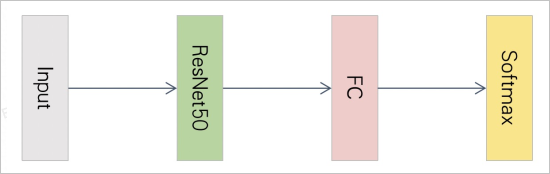In a classification task that has a large number of categories, weight values on the FC node cannot be effectively communicated when data parallelism is implemented. When the number of categories increases, the GPU memory is insufficient for weight values of the model. In this case, you must implement operator splitting to optimize model training in a distributed manner, as shown in the following figure.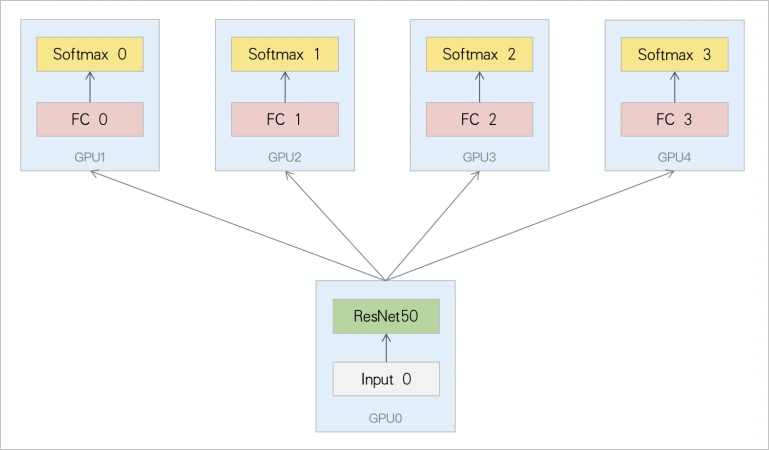Weight values on the FC node are split by column, as shown in the following figure. The output of the ResNet50 model is sent to each shard of the FC node. Each shard calculates only the output that it receives. After the output logits are obtained, computing is separately performed for each Softmax. This requires cross-GPU communication to obtain the global maximum logits and the sum of each row. For more information about the distributed computing for a classification task, see Perform operator splitting in distributed training for large-scale classification.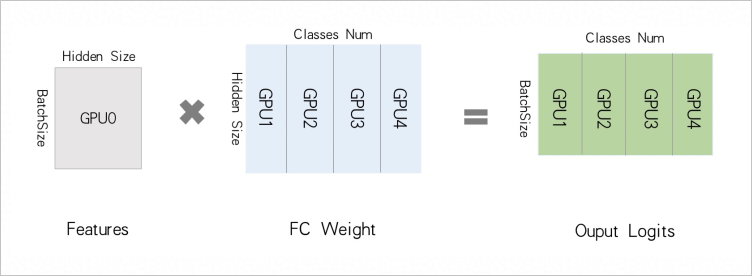• Operator splitting in Whale
To implement operator splitting in Whale, you must configure the cluster layout and add the code for operator splitting to the scope code. In this example, the ResNet50 model is used. The following code provides an example on operator splitting. For more information about the complete code of operator splitting for large-scale classification, visit large_scale_classification.py.
``````import whale as wh
cluster = wh.cluster()

with cluster:
with wh.replica():
features = ResNet50(inputs)

with wh.split():
logits = FC(features)
predictions = Softmax(logits)``````